+
Set Notation and Interval Notation
Solving Linear Equations and Inequalities
0
of 0 possible points

# Set Notation and Interval Notation

Author: Sophia Tutorial
##### Description:

Identify the correct notation for a given number line

(more)

Sophia’s self-paced online courses are a great way to save time and money as you earn credits eligible for transfer to many different colleges and universities.*

No credit card required

37 Sophia partners guarantee credit transfer.

299 Institutions have accepted or given pre-approval for credit transfer.

* The American Council on Education's College Credit Recommendation Service (ACE Credit®) has evaluated and recommended college credit for 33 of Sophia’s online courses. Many different colleges and universities consider ACE CREDIT recommendations in determining the applicability to their course and degree programs.

Tutorial
what's covered
1. Set Notation
2. Interval Notation
3. Writing a Solution Set from a Number Line

# 1. Set Notation

When looking at number lines with a range of highlighted values, we can write the range of values a couple of different ways: in a format called set notation, and in a format called interval notation. In set notation, we define the range of values as set of numbers, and we use curly braces to define the set, with a description of what is to be included in the set. Interval notation, on the other hand, describes an interval of values represented by the number line.

Let's examine the number line below.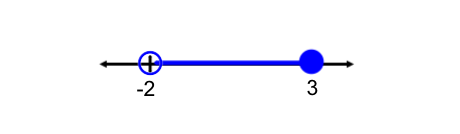We see that the highlighted values range from -2 to +3. Be sure to examine what kind of circles are used on the number line. Open circles indicate that the exact value of -2 is not included in the set, and the closed circle indicates that +3 is included in our set. This is going to have important implications on what kind of inequality symbols we use in set notation.

In set notation, this is written as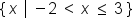This is read as, "all x-values, such that x is between (but not including) -2, and 3 (including 3).

big idea
Set notation uses curly braces to define the number line solution as a set of values. Open circles corresponding to the strict inequalities < and >, while closed circles correspond to non-strict inequalities ≤ and ≥. In set notation, the vertical bar is read, "such that..."

# 2. Interval Notation

In interval notation, we define specific intervals on the number line, and enclose the range of values using either square brackets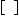or parenthesis,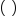. Square brackets correspond to the closed circles on the number line, or non-strict inequalities ≤ and ≥. The parentheses are used when there are open circles on the number line, and correspond to the strict inequalities < and >. Also note that parentheses, never square brackets, are always used with the infinities. This is because positive and negative infinity are not concretely defined as numbers.

We can write the same number line as above using interval notation. Here is our number line with a highlighted range of values.As before, we see that the highlighted range is from -2 to +3. We do not include the exact value of -2, but we do include the exact value of 3. So we will use parentheses to enclose -2, but square braces to enclose 3.

In interval notation, this is written as: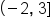, or left parenthesis negative 2 comma 3 right square bracket. This means that the solution on the number line is in the interval from (but not including) -2 up to, and including, 3.

big idea
Interval notation describes an range of values from a starting point to a stopping point. We use either square braces or parentheses, depending on if we are including or excluding exact values. Square bracesare used to include exact values, and parenthesesare used to exclude exact values. We always use parentheses with the infinities.

# 3. Writing a Solution in Interval and Set Notation

Let's practice writing in set and interval notation, using number line solutions. Examine the number line below:We notice that all values from 2 to positive infinity are highlighted. In set notation, we would write this as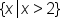. Note that our inequality symbol does not include the exact value of 2.

In interval notation, this is written as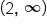. Note that we use a parenthesis to the left of 2, and also a parenthesis to the right of infinity.

Let's look at a more complicated example: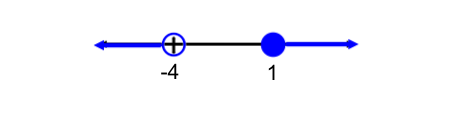This number line has two ranges highlighted. How do we write this in set notation and interval notation? We have two inequality statements to include in our set. First, we have x < -4. We also have x ≥ 1. To write this in set notation, we write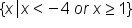. We use the connecting word "or" because values that fit within either inequality statement will fit the number line solution.

In interval notation, we define two intervals: the first interval is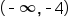. and the second interval is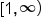. To accept both intervals as solutions for x, we use the symbol for union, U, to connect the two intervals. To complete our solution in interval notation, we have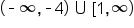.

summary
In set notation, we define the range of values as set of numbers and use curly braces to define the set, with a description of what is to be included in the set. Interval notation, on the other hand, describes an interval of values represented by the number line and use parentheses or brackets. When writing a solution in interval and set notation, it is important to pay attention to the different inequality signs and corresponding symbols.

Rating